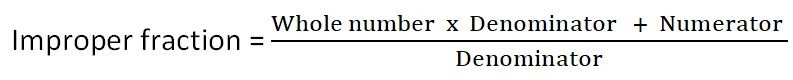# How to convert the mixed fraction into an improper fraction?

We can convert a mixed fraction into an improper fraction using the following formula:For example, consider $8\frac{4}{7}$.

Here Whole number = 8, Denominator = 7, Numerator = 4.

Substitute in the above formula

$8\frac{4}{7}=\frac{(8\times7)+4}{7}=\frac{60}{7}$.

Updated on: 10-Oct-2022

71 Views